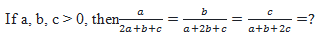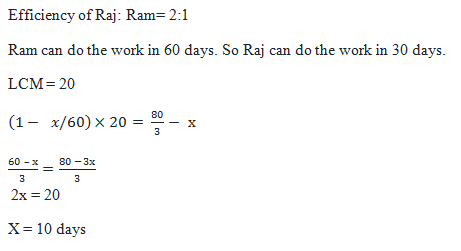# SSC CGL 2018 Practice Test Papers | Quantitative Aptitude (Day-41)

Dear Aspirants, Here we have given the Important SSC CGL Exam 2018 Practice Test Papers. Candidates those who are preparing for SSC CGL 2018 can practice these questions to get more confidence to Crack SSC CGL 2018 Examination.

[WpProQuiz 3362]

Click “Start Quiz” to attend these Questions and view Explanation

1) Three types of pulses A, B and C were used in a hotel in the ratio of 3: 1: 6 for preparing dinner. The cost of pulses A, B and C per kg is Rs. 80, Rs. 54 and Rs. 72 respectively. The average cost of the three pulses A, B and C per kg was:

a) 75

b) 64

c) 77.2

d) 72.6

2) Raj is twice as efficient as Ram and Ram can do a piece of work in 60 days. Ram started the work and after a few days Raj joined him. The total work completed in 80/3 days. For how many days did Ram work alone from starting of the work?

a) 12

b) 10

c) 15

d) 20

3)a) ½

b) 1/3

c) ¼

d) 1/8

4)  A bus is travelling at a speed of 55 km/h and it overtakes another bus which travelling at a speed of 19 km/h in 12 seconds. If the ratio of the lengths of the buses is 7: 5, then, find out the length of the shorter bus?

a) 50 m
b) 18 m
c) 26 m
d) 25 m

5) The product of the ages of M and N is 805 years. Three years ago, N’s age was 5/8 of the age of M. What is N’s present age?

a) 20 years
b) 21 years
c) 48 years
d) 23 years

6) The sum of area of a circle and a square is equal to 2788 cm2. The diameter of a circle is 56 cm. What is the ratio of the perimeter of square to the circumference of circle?

a) 5: 12
b) 15: 28
c) 12: 19
d) 9: 22

7) Train A can cross train B, when running in the same direction, in 48 seconds, while train A cross train B when running in opposite direction in 36 seconds. Find the ratio of speed of train A to speed of train B.

a) 7 : 1
b) 5 : 3
c) 3 : 1
d) None of these

8) A car travels along four sides of square at speeds of 150 km/h, 200 km/h, 300 km/h and 600 km/h. Find the average speed of the car.

a) 200 km/h
b) 140 km/h
c) 240 km/h
d) 320 km/h

9) What will be the percentage profit after selling an article at label price if there is loss of 30% when the article is sold at 1/3 of the label price ?

a) 80%

b) 90%

c) 110%

d) 150%

10) If x2x 1 =0 find Value of x32x 1 =?

a) 0

b) 1

c) 2

d) 4

Assume that 3 kg of pulse of type A, 1 kg of B and 6 kg of C are used in the hotel

Total Cost= 3×80+1×54+6×72 = 726

Average cost= 726(3+1+6) = 72.6Substitute a = 1, b = 1 and c = 1

Relative Speed = 55 19 = 36 km/h = 36 × 5/18 = 10 m/s

First bus replaces the bus in 12 seconds

Distance covered = 12×10 = 120 m

12 —- 120

5 —— ?

? = 50 m

MN = 805

M = 805/N  —– (i)

N – 3 = 5/8 (M – 3)

8N – 5M = 9 —– (ii)

Substitute (i) in (ii)

8N – 5(805/N) = 9

8N2 – 4025 = 9N

N = 23

Diameter of circle = 56

Radius of circle, r = 28

22/7×28×28 = 2464

Area of Square = 324

a2 = 324

Side of a Square, a = 18

Perimeter of Square: Circumference of Circle = 4a: 2πr

=72: (2×22/7×28)

=72: 176

= 9: 22

48(s1-s2)=36(s1+s2)

48s1-48s2=36s1+36s2

12s1=84s2

S1:s2=84:12=7:1

Let the side of a square =x

Perimeter of Square= 4x

Speed = Distance/ Time

=4x/(x/150+x/200+x/300+x/600)

=4x/(10x/600)

=240 kmph

Let Label price be Rs. 100.

It is sold at 30% loss i.e Rs. 70

1/3 of Label Price = 70

Label Price = 70× 3= 210

Profit %= 110/100 ×100

= 110% profit

Given x2 – x – 1

=>x2 – 1 = x

x2 – 1 – 1 = x – 1

x2 – 2 = x – 1 …..(i)

Again, x2 – x – 1 = 0

x2 – x = 1 ….(ii)

Value of x3 – 2x + 1

= x(x2 – 2) + 1

= x(x – 1) + 1 [from i]

= x2 – x + 1

= 1 + 1 [from ii]

= 2